# Fourier transform facts for kids

Kids Encyclopedia Facts

The Fourier transform is a mathematical function that can be used to find the base frequencies that make up a signal or wave. For example, if a chord is played, the sound wave of the chord can be fed into a Fourier transform to find the notes that the chord is made from. The output of a Fourier transform is sometimes called a frequency spectrum or distribution because it displays a spectrum of the frequencies of the input. This function has many uses in cryptography, oceanography, machine learning, radiology, quantum physics as well as sound design and visualization.

The Fourier transform of a function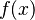$f(x)$ is given by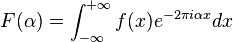$F(\alpha)=\int_{-\infty}^{+\infty} f(x) e^{-2 \pi i \alpha x} dx$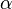$\alpha$ is a frequency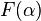$F(\alpha)$ is the Fourier transform function and returns a value representing how prevalent frequency$\alpha$ is in the original signal.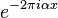$e^{-2\pi i \alpha x}$Represents wrapping the input function$f(x)$ around the origin in the complex plane at some frequency$\alpha$

The inverse Fourier transform is given by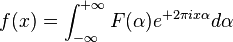$f(x)=\int_{-\infty}^{+\infty} F(\alpha) e^{+2 \pi i x \alpha} d \alpha$

A Fourier transform shows what frequencies are in a signal. For example, consider a sound wave which contains three different musical notes: A, B, and C. Making a graph of the Fourier transform of this sound wave (with the frequency on the x-axis and the intensity on the y-axis) will show a peak at each frequency which corresponds with one of the musical notes.

Many signals can be created by adding together cosines and sines with varying amplitudes and frequencies. The Fourier transform plots the amplitudes and phases of these cosines and sines against their respective frequencies.

Fourier transforms are important because many signals make more sense when their frequencies are separated. In the audio example above, looking at the signal with respect to time does not make it obvious that the notes A, B, and C are in the signal. Many systems do different things to different frequencies, so these kinds of systems can be described by what they do to each frequency. An example of this is a filter which blocks high frequencies.

Calculating a Fourier transform requires understanding of integration and imaginary numbers. Computers are usually used to calculate Fourier transforms of anything but the simplest signals. The Fast Fourier Transform is a method computers use to quickly calculate a Fourier transform.

## Images for kidsFourier transform Facts for Kids. Kiddle Encyclopedia.